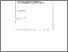# An Adaptive Method for Minimizing a Sum of Squares of Nonlinear Functions

Nazareth, J.L. (1983). An Adaptive Method for Minimizing a Sum of Squares of Nonlinear Functions. IIASA Working Paper. IIASA, Laxenburg, Austria: WP-83-099Preview Text WP-83-099.pdf Download (545kB) | Preview

## Abstract

The Gauss-Newton and the Levenberg-Marquardt algorithms for solving nonlinear least squares problems, minimize F(x) = sum_i=1^m (f_i(x))^2 for x in R^n, are both based upon the premise that one term in the Hessian of F(x) dominates its other terms, and that the Hessian may be approximated by this dominant term J^T J, where J_ij = ( delta f_i / delta x_j ). We are motivated here by the need for an algorithm which works well when applied to problems for which this premise is substantially violated, and is yet able to take advantage of situations where the premise holds. We describe and justify a method for approximating the Hessian of F ( x ) which uses a convex combination of J^T J and a matrix obtained by making quasi-Newton updates. In order to evaluate the usefulness of this idea, we construct a nonlinear least squares algorithm which uses this Hessian approximation, and report test results obtained by applying it to a set of test problems. A merit of our approach is that it demonstrates how a single adaptive algorithm can be used to efficiently solve unconstrained nonlinear optimization problems (whose Hessians have no particular structure), small residual and large residual, nonlinear least squares problems. Our paper can also be looked upon as an investigation for one problem area, of the following more general question: how can one combine two different Hessian approximations (or model functions) which are simultaneously available? The technique suggested here may thus be more widely applicable and may be of use, for example, when minimizing functions which are only partly composed of sums of squares arising in penalty function methods.

Item Type: Monograph (IIASA Working Paper) System and Decision Sciences - Core (SDS) IIASA Import 15 Jan 2016 01:53 27 Aug 2021 17:11 https://pure.iiasa.ac.at/2213View Item